Technical Report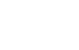Damping materials and their performance measurement
(page11-2)

29. Measurement of loss factor for distortion of damping alloys

When the loss factor of damping alloys is measured, the amplitude dependent occurs due to the damping generating mechanism. This characteristic is not found in viscoelastic materials which are not affected by vibration amplitude. For this measurement, it is necessary to attach a strain gauge at the center position during central exciting and create a graph using the strain amplitude as a parameter. It is also an efficient measurement to replace the tip amplitude (measured with a laser vibrometer) with the strain amplitude at the center position since it is troublesome to attach strain gauges on all measurement specimens, problem of S/N rate. The block diagram is shown as follow:

 The system configuration for the measurement of loss factor for distortion of damping alloys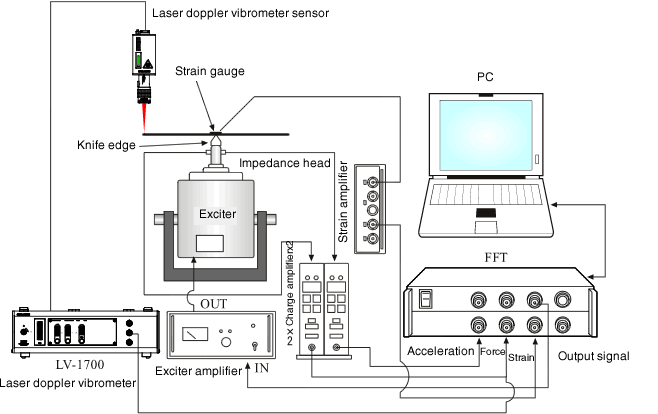Fig. 1

The graph below shows that the tip amplitude to the same center strain amplitude lines are drawn even if the materials have the same dimensions, thicknesses but different Young’s modulus (7 kinds of Young’s modulus).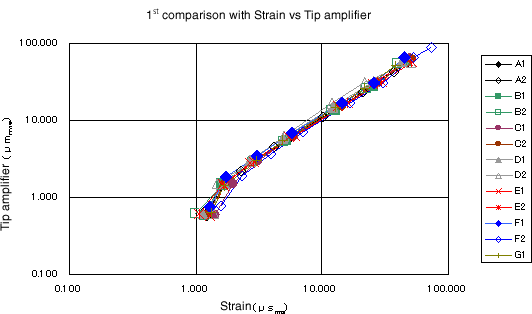Fig. 2

The measurement method of loss factor is one that changes the amplitude gradually, and obtains from the mechanical impedance with the half-width method (amplitude rate 100: 1), and another that vibrates via impulse or tone burst wave with resonance frequency and obtains from damping at time axis via logarithmic decrement.
The following 2 figures are obtained from the second method as mentioned above.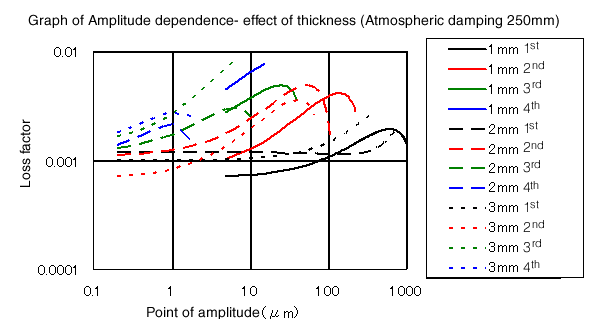Fig. 3

It shows that it depends not only on amplitude but also on material thickness. The figure shows material comparison.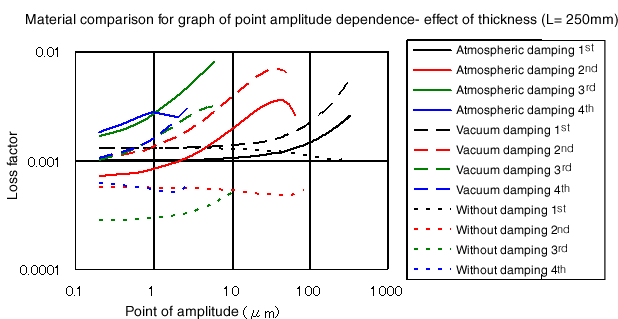Fig. 4

The figure below is a graph with the horizontal axis replaced by the strain amplitude (The data is different from the above figure).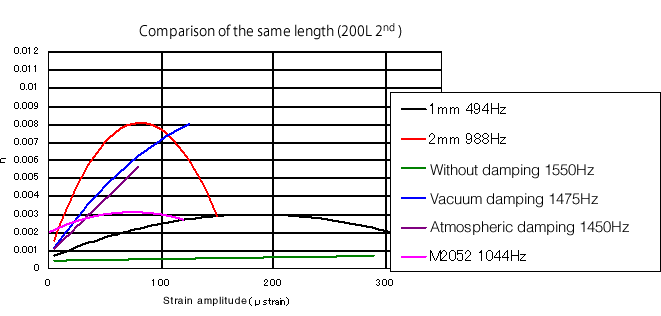Fig. 5

As shown in the figure below, it is easy to read a contour diagram in which the horizontal is amplitude strain, the vertical is frequency, and the loss factor is perpendicular to the paper (Z direction).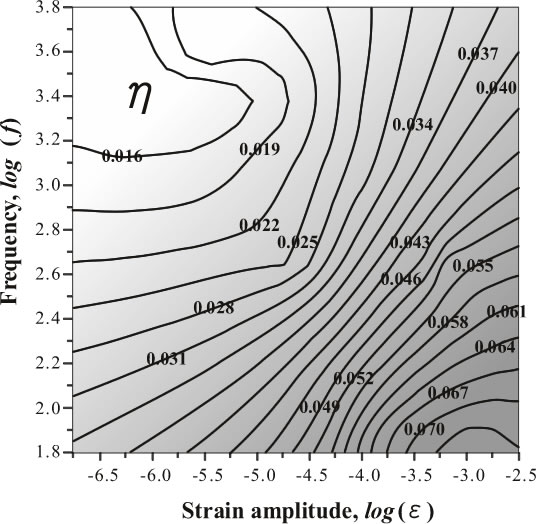Fig. 6